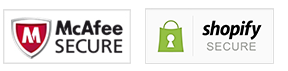# Fantasy Horror Silk Cloth 3D Wallpaper

Regular price \$39.95 Sale price

\$59.99##### Not Sold In Stores!
###### 100% Safe and Secure Checkout!

Function:

• Smoke-Proof
• Waterproof
• Moisture-Proof
• Mould-Proof
• Soundproof
• Sound-Absorbing
• Heat Insulation
Feature:
• Water Resistant
• Formaldehyde-free
• Mildew Resistant
• Extra Thick
• Environment Friendly

Material: Silk wallpaper
Surface Treatment: Printed

Mural wallpaper price: \$ / square meter. Not rolls.

square meter = 1pcs.   2 square meter=2pcs.   3 square meter =3pcs ...

Width x height = square meters = Order Quantity

Quantity: 1 square meter = 140cm (W) x 70cm (H) (4'3 "x 2'3") ft

Quantity: 2 square meter = 200cm (W) x 100cm (H) (6'5 "x 3'2") ft

Quantity: 3 square meter = 208cm (W) x 146cm (H) (6'8 "x 4'8") ft

Quantity: 4 square meter = 200cm (W) x 200cm (H) (6'5 '' x 6'5 '') ft

Quantity: 5 square meter = 250cm (W) x 200cm (H) (7'6 '' x6'5 '') ft

Quantity: 6 square meter = 300cm (W) x 200cm (H) (9'8 '' x 6'5 '') ft

Quantity: 7 square meter = 300cm (W) x 250cm (H) (9'8 "x 8'2") ft

Quantity: 8 square meter = 400cm (W) x 200cm (H) (13'6 "x 6'5") ft

Quantity: 9 square meter = 350cm (W) x 260cm (H) (11'4 "x 8'7") ft

Quantity: 10 square meter = 360cm (W) x 280cm (H) (11'8 "x 9'2") ft

Quantity: 11 square meter =  420cm(W) x 260cm(H) (13'9'' x 8'6'') ft

Quantity: 12 square meter = 400cm (W) x 300cm (H) (13'6 "x 9'8") ft

Quantity: 13 square meter = 460cm(W) x 280cm(H) (15'1'' x 9'2'') ft

Quantity: 14 square meter = 480cm(W) x 290cm(H) (15'9'' x 9'6'') ft

Quantity: 15 square meter = 500cm(W) x 300cm(H) (16'5'' x 9'10'') ft

Quantity: 16 square meter = 500cm (W) x 320cm (H) (16'4 "x 10'5") ft
OUR GUARANTEE
##### 100% satisfaction guarantee / 60 day money back guarantee. If for ANY reason you are not happy with our product, simply return it for a full refund. Your purchase is Safe and Secure with us.We have 24/7 customer service via Email Support or Phone.Please feel free to contact us at any time!Email: support@skullflow.comPhone: +1 (571) 200-5175